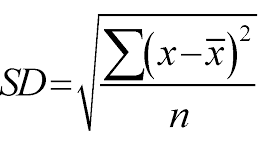Name:______________________________________

# Calculate Standard Deviation with Hair1. Use a meter stick to measure the length of hair for members of your class. Record each length in the space below. To simplify, you may wish to only choose girls, or boys. You should have at least 5 data points. The variable of interest (Xi) is hair length.

2. What is the average length of hair in the class based on your sample?

This is x̄ .

3. What is the average distance of hair length from the mean? To calculate the Variance (σ), take each difference, square it, and then average the result:

4. The square root of your Variance (σ) is the standard deviation.

### Analysis

5. What if the standard deviation for hair length for your sample is 0. What does this mean?

6. If you added a new person to your data set who has hair that is equal to the average length for your group, what impact will this have on your standard deviation?

7. Why would scientists care about standard deviation when they are looking at sets of data?